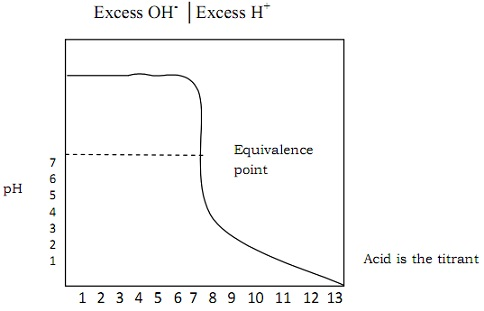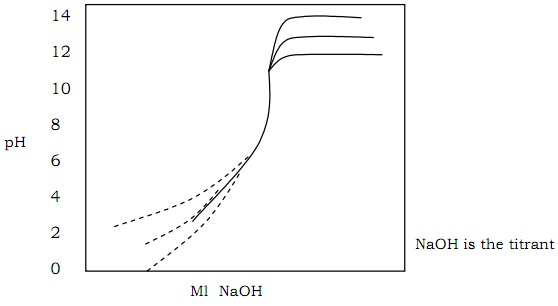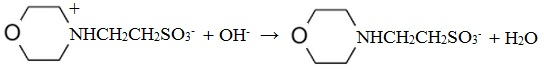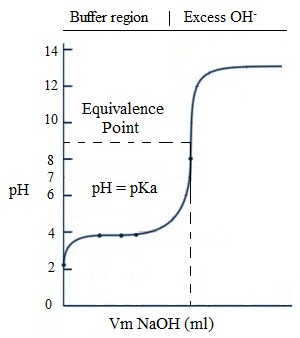#### Acid-Base Titration, Chemistry tutorial

Introduction:

Acid-base titration is one of the kinds of volumetric analyses. It is most generally employed all through the realm of chemical analysis. Through the use of titration curve, both the acidic and basic component of a material (or sample) can be determined.

Acid-base Titration:

It is a volumetric analytical process that relates acid and base stoichiometrically till reaction is completed. It is a very simple, reproducible and precise analytical method.

Acids and bases have been illustrated via different theories notably which are:

1) Arrhenius theory that illustrates an acid as any substance which ionizes partially or fully in water to provide hydrogen ion, whereas base is any substance that ionizes partially or fully in water to provide hydroxyl ions.

2) Bronsted-Lowry theory explains an acid as a substance which can donate a proton and a base as any substance which can accept a proton. There is a medium (or solvent) essential for the titration reacting to take place that can be aqueous or non-aqueous.

Classification of Solvents:

The solvents might be categorized into three groups based on some principles.

1) Amphiprotic: Those solvents which have both the basic and acidic properties example: water and ethanol. These kinds of solvents can ionize.

2) Aprotic: Those solvents which are neither appreciably acidic nor basic. They are weakly polar in nature example: benzene tetrachloride.

3) Basic but not acidic: Such solvents are extremely weak bases. They are non-ionisable example: ether and ketone.

Monitoring pH changes:

For the study of different acid-base titration, to be comprehensive and goal oriented, the study would supervise trends of change in pH as titrant is added to the analyte. These phases cover:

• Before equivalence point
• At equivalence point
• After equivalence point

Titration of Strong Acid against Strong Bases:

The reaction is neutralization. This kind of acid-base titration is for all time a strong acid on a strong base.

The first step is to write down a comprehensive ionic equation of the reaction.

Illustration: Titrating HCl against NaOH

The exercise in the system is H+ + Cl- + Na+ + OH → H2O + Na+ + Cl-

The ionic equation is: H+ + OH+ → H2O

This is or else termed as titration reaction. The equation can as well assist in finding out computing the composition and pH after each addition of the titrant.

Vx (ml) (Molarity of x) = Vy (ml) (Molarity of y)

Vx = Vy My/mx

Here, 'x' is for acid and 'y' is for the base

At 'Vx' the equivalence point is reached. Before this Vx point, there will be surplus of OH and after the Vx point; there will be surplus of H+. Thus, in plotting titration curve pH versus the volume (y) of the titrant (x), there are three variable areas, viz:

a) Before reaching the equivalence point, pH is found out by the surplus of OH in the solution.

b) At equivalence point, the H+ is just adequate to fully react with all OH. Therefore, the pH is determined via dissociation of water, whereas KW = 10-14

Therefore, the reaction goes to completion.

c) After reaching the equivalence point, pH is found out by the surplus H+ in the solution.Fig: The titration curve

The magnitude of the break (that is, end point) based on the concentration of both titrant and analyte.Fig: Titration curve

Curve 1: 0.1m HCl against 0.1 m NaOH

Curve 2: 0.01 HCl against 0.01m NaOH

Curve 3: 0.001 HCl against 0.01m NaOH

Titration of weak Acids against Strong Bases:

The titration reaction is neutralization. Strong base is the titrant whereas the weak acid is the analyte.

The first step is to write the ionic equation.

If we are titrating MES against NaOH

MES 2 → (N-morphline) ethane-sulphonic acid. This is a weak acid with pKa = 6.15Fig: Titration Equation

From the reaction:

Vm (ml) (molarity Mm) = Vn (ml) (Molarity Mn)

Vm = Vn Mn/Mm

It is useful to compute Vm required reaching the equivalence.

Here m = strong base (titrant) and n = weak acid (analyte)

The titration computation is then divided into four phases which is as well reflected in the titration curve.

1) Before any base is added, the solution includes just HA in water. This is a weak acid problem in which pH is found out by the equilibrium.

HA ↔ (Ka) ↔ H+ + A-

2) From the first addition of NaOH till before the equivalence point, there exist a mixture of unreacted HA and the A- produced by the reaction. Henderson-Hasselbalch equation can be employed to determine the pH.

Hasselbalch equation is the equation that expresses pH of a buffer solution as a function of the concentration of the weak acid or base and the salt component of the buffer system.

Hasselbalch equation: pH = pKa + log [A-]/[HA]

3) At equivalence point 'all' of the HA has been transformed to A-. The problem is similar as if the solution had been made by just dissolving A- in water. pH can be found out by the reaction.

A- + H2O ↔ HA + OH-

4) Beyond the equivalence point, surplus NaOH is being added to the solution of A- to a good approximation the pH is found out by strong base. We compute the pH as if we simply add surplus NaOH to the water.Fig: Titration curve-weak acid and strong base

Before the titration reaction began at all, we have just weak acid in the medium acid and therefore pH is computed as for weak acid. At the commencement of titration, a few HA is transformed to A- and therefore buffer system is set up. As the titration carry on, the pH slowly raise as the ratio of A- : HA changes.

At the mid-point of the titration [OAc] = [HOAc] and pH is equivalent to pKa. The pH of equivalence point will be alkaline.

The weaker the acid (that is, the smaller Ka), the longer the positive Kb of the salt and the more alkaline is the equivalence point.

Titration of Weak Base against Strong Acids:

It is as well a neutralization reaction. This is just the opposite of the case of weak acid against the strong Base.

The titration reaction is: Β + H+ → BH+

The reaction goes to completion after each and every addition of acid. There are as well four phases described in titration curve.

a) Before acid is added, the solution consists of just the weak base in water.

The pH is finding out by the Kb reaction.

B + H2O ↔ BH + OH-

b) After the beginning of titration up to equivalence point there is a mixture of B and BH+. That is Buffer system is set up. Kb can simply be found out from the titration curve.

c) At the equivalence point. B has been transformed into BH+, a weak acid. The pH is computed by the consideration of dissociation of acid BH+

BH+ ↔ (Kb) ↔ B + H+    Ka = Kw/Kb

The  formal  concentration  of  BH+,  F'  is  not  similar  as  the  original  formal concentration  of B,  due to some dilution which has occurred. As the solution includes BH+ at the equivalence point, it is acidic. The pH at the equivalence point should be beneath 7.

d) After the equivalence point, there is surplus of H+ in the solution.Fig: Titration curve-Weak base and strong acids

Titration of Polyprotic Systems:

There are some bases which hydrolyze in more than one step, thus having more than one dissociation constant. Usually, the principle developed for the titration of monoprotic systems eagerly applies to them.

For illustration, consider titration of base (B) against Acid (H) in which the base is dibasic.

The titration equation:

B + H → BH+       ...... (a)

BH+ + H+ → BH22+...... (b)

The titration curve illustrates reasonable sharp breaks at both the equivalence points.

From equation (a):

Vb x nb = Va x na

Here 'b' is for the base and 'a' is for the acid

Va = (Vb x na)/Ma

The volume of the acid needed to reach first equivalence point is Va1

Going by equation (b), the volume of acid to reach second equivalence point is 2 x VaiFig: Titration of Polyprotic Systems

Point 'C' and 'E' are the two equivalence points whereas point 'B' and 'D' are the half- neutralization points whose values equivalent to pKa2 as pK1 correspondingly. The titration curve is in the given stages.

a) Point A: Before any acid is added, the solution consists of just B, a weak base whose pH can be found as follows.

B + H2O → (Kb) → B+ + OH-

y - x                        x        x

Here, y is the original volume of B in the system

[H+] = (Kw/x) = pH

b) Point B: Between the point A and C, we encompass buffer system established having B and BH+. B is half way to equivalence point. Therefore, [B] = [BH+], pH is computed by employing Henderson-Hasselbalch for weak acid whose dissociation constant is Ka2 for BH22+

Ka2 = Kw/Kb1

To compute pH at B:

pH = pKa + log [B]/[BH+]

c) Point C: This is the first equivalence point. B has been transformed to BH+, the intermediate form of diprotic acid BH22+.

[H+] = √[(K1K2F + K1Kw)/(K1 + F)]

Here, F is the formal concentration of BH+

pH = 1/2 [PK1 + PK2]

d) Point D: At any point between C and E, we can take the solution to be buffer having BH+ and BH22+

pH = pKa1 + log [BH+]/BH22+

e) Point E: Point E is the second equivalence point at which solution is formerly similar. The pH is found out by the acid dissociation reaction of BH22+.

BH22+ ↔ BH+ + H+

F-x           x         x

Detecting the End Point with Indicator:

The use of an acid-base indicator to determine end point is a more convenient process. It comprises adding small amount of acid-base indicator, itself an acid or base, whose different protonated species encompass different colors.

The colour of the ionized form is dissimilar from the unionized form. One form might be colored; the other form might be colourless.

HIn ↔ H+ + In-

(Red)  (Blue)

The unionized form is red whereas the ionized form is blue. Then the Henderson-Hasselbalch equation provides:

pH = pKIn + log [ln-]/[HIn]

Note that as the indicator is a weak acid or base, the amount added must be kept minimal in such a way that it doesn't contribute appreciably to the pH. Furthermore, the smaller the quantity of the indicator added, the sharper the colour alters.

There are other methods although not as simple as the use of indicator, through which end point can be detected. These comprise:

• The use of pH electrode
• The use of derivatives
• The use of gram plot

Tutorsglobe: A way to secure high grade in your curriculum (Online Tutoring)